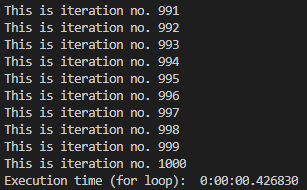# How to Calculate the Execution Time of a Small Python Program

Hello everyone, In this tutorial, we’ll be going to learn how we can calculate the execution time of a Python program. For this, we’ll be using a very handy and useful Python library named ‘DATETIME‘. After this tutorial, we will be able to know which code for a specific functionality runs faster than others. As it is an inbuilt library that comes with Python, so we do not require to install it.

Implementation Overview

On the basis of the implementation below, we’ll see how we can write code.

```initial_time = Time before starting the code
"""

"""
final_time = Time after code the code completes
Execution_time = final_time-initial_time```

## How to Calculate the Execution Time of a Python Code

We will write a code to print 1000 lines using For Loop and While loop and we’ll see who works faster.

```from datetime import datetime

init_time = datetime.now()
for i in range(1000):
print(f"This is iteration no. {i+1}")

fin_time = datetime.now()

print("Execution time (for loop): ", (fin_time-init_time))```
1. First, we import the datetime module from datetime library which has a class method named now() that we will be using.
2. Then we initialize a variable init_time that holds the values of date and time before the code runs.
3. Using for loop we have now printed 1000 lines.
4. Similar to step 2, now we have an initialize variable fin_time that holds the value of time after the loop execution ends.
5. Difference between the Final time and Initial time is the Execution Time of our code.

#### The output will look like this.The output represents time as hours: minutes: seconds. microseconds. This is how we can calculate execution time. Lets us try the same code using While Loop and see the difference.

```init_time_while = datetime.now()
j=0
while j<1000:
print(f"This is iteration no. {j+1}")
j +=1
fin_time_while = datetime.now()
print("Execution time (while loop): ", (fin_time_while-init_time_while))```

Everything is the same as we have discussed for the For loop just we have written a while equivalent code. We want you to try out the above code and see the difference between both using the code below.

```overall_time = (fin_time_while-init_time)
loops_time_difference = ((fin_time-init_time)-(fin_time_while-init_time_while))

print("Difference: ", loops_time_difference)```

Note:

• Execution time highly affected by the current environment and conditions of your PC like Background Processes Running, Current Load, Temperature of your system, Memory usage, Processor used etc many. So try to run them in as much the same environment as possible.
• For the code above we may get short difference between both but for the functionality like above they are considered to have the same performance and both have their own advantages over others in certain conditions.
• Because we have used datetime module we will get both the date and the time. Moreover, there are more ways to find execution time but this method is more accurate than others.
• This method may not be useful during many function calls and loops in the same program or simply for larger programs.

We hope you like this tutorial and if you have any doubts feel free to leave a comment below.

You may like to learn

How to convert .py to .exe

Python for loop decrementing index

Python Program to reverse digits of a given number